# PSAT Math : How to find the length of a diagonal of a polygon

## Example Questions

### Example Question #105 : Plane Geometry

Regular Octagon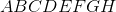has sidelength 1.

Give the length of diagonal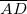.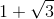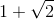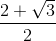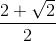Explanation:

The trick is to construct segments perpendicular tofromand, calling the points of intersectionand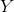respectively.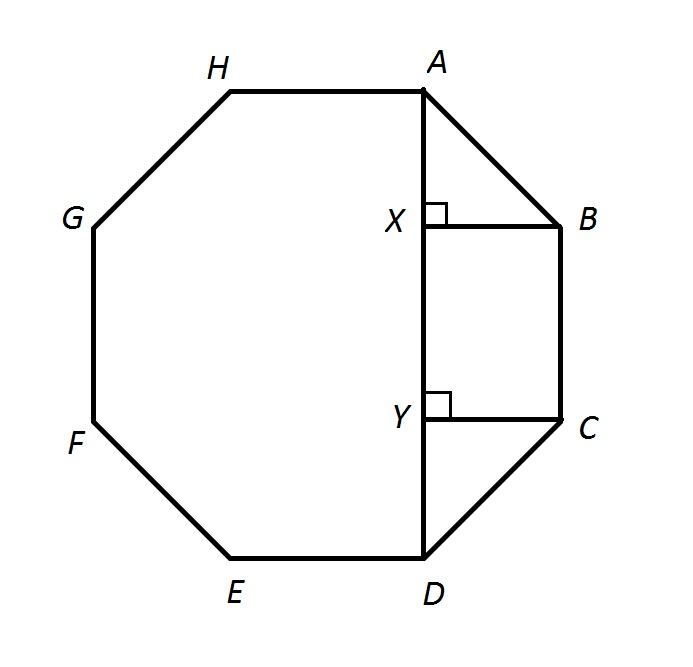Each interior angle of a regular octagon measures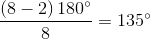,

and by symmetry,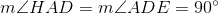,

so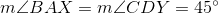.

This makes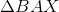and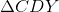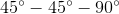triangles.

Since their hypotenuses are sides of the octagon with length 1, then their legs - in particular,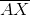and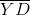- have length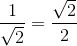Also, since a rectangle was formed when the perpendiculars were drawn,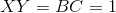.

The length of diagonalis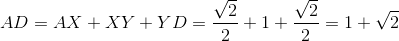.

### Example Question #1 : How To Find The Length Of A Diagonal Of A Polygon

Regular Polygon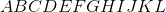(a twelve-sided polygon, or dodecagon) has sidelength 1.

Give the length of diagonalto the nearest tenth.Explanation:

The trick is to construct segments perpendicular tofromand, calling the points of intersectionandrespectively.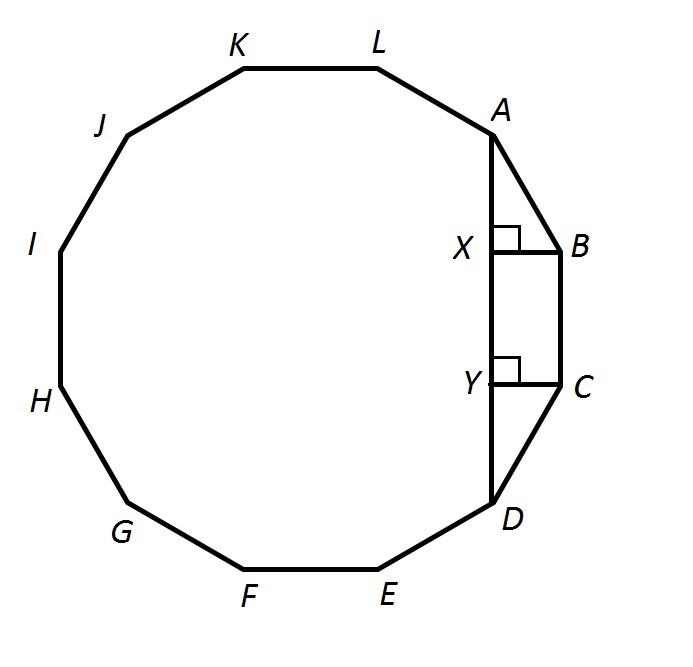Each interior angle of a regular dodecagon measures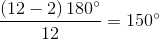.

Since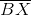and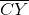are perpendicular to, it can be shown via symmetry that they are also perpendicular to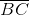. Therefore,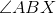and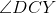both measureand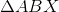and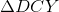are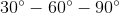triangles with long legsand. Since their hypotenuses are sides of the dodecagon and therefore have length 1,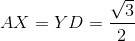.

Also, since Quadrilateral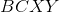is a rectangle,.

The length of diagonalis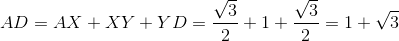.